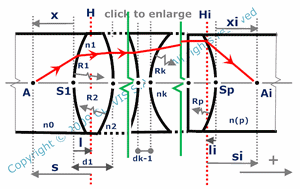and orgeometrical optics
paraxial conjugation

# conjugation by a combination of plane and spherical refracting surfaces

 number of surfacesRk : radius of curvature of surface number k nk : refraction index of the medium after surface number k dk : distance from the vertex of surface number k to the vertex of surface number k+1 x : distance from the vertex of the first surface to the object point l : distance from the vertex of the first surface to the primary principal plane of the combination li : distance from the vertex of the last surface to the secondary principal plane of the combination s : distance from the primary principal plane of the combination to the object point si : distance from the secondary principal plane of the combination to the image point xi : distance from the vertex of the last surface to the image point m : magnification of the combination

Note
This calculation page can not detect all unrealistic inputs.